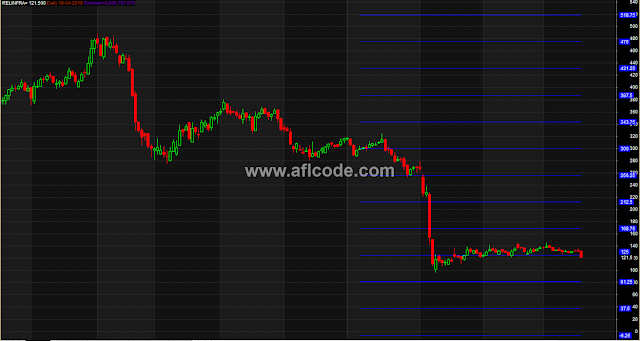### Static Support Resistance Horizontal LinesStatic Support Resistance Horizontal Lines
```//www.aflcode.com

_SECTION_BEGIN(" Support and Resistance ");

GraphXSpace=1; MaxGraph=15;
Period=Param("MM_Period", 70, 4, 256, 1) ;

Graph1Color=6; Graph2Color=6; Graph3Color=6; Graph4Color=6;
Graph5Color=6; Graph6Color=6; Graph7Color=6; Graph8Color=6;
Graph9Color=6; Graph10Color=6; Graph11Color=6;Graph12Color=6;
Graph13Color=6;

Graph0=Close; Graph0Color=1; Graph0Style=64;

Graph1Style=1; Graph2Style=1; Graph3Style=1; Graph4Style=1;
Graph5Style=1; Graph6Style=1; Graph7Style=1; Graph8Style=1;
Graph9Style=1; Graph10Style=1; Graph11Style=4; Graph12Style=1;
Graph12Style=1;

PER = Period;
BarNR = LastValue(Cum(1)) ;
TimeFrame = BarNR - PER ;
TF = TimeFrame ;

Lo = LastValue(ValueWhen(Cross(Cum(1), TF), LLV(Min(H,L),PER),1)) ;
Hi = LastValue(ValueWhen(Cross(Cum(1), TF), HHV(Max(H,L),PER),1)) ;

Lo = LastValue( LLV(Min(H,L),PER ),1) ;
Hi = LastValue( HHV(Max(H,L),PER ),1) ;

Fractal = IIf(Hi<=250,100, IIf(Hi<=2500,1000, IIf(Hi<=25000,10000, IIf(Hi<=250000,100000, 0 )))) ;
int_Log = int( log(Fractal/(Hi-Lo))/log(2) ) ;
Oct = Fractal * ( exp(ln(1/2)* int_Log ) ) ;

Min_1 = int( Lo / Oct ) * Oct ;

Max_1 = IIf( (Min_1 + Oct) > Hi, Min_1 + Oct, Min_1 + 2*Oct ) ;

Min_2 = IIf( (Min_1 + 1/8*(Max_1-Min_1)) <= Lo AND Hi <= (Max_1 - 1/8*(Max_1-Min_1)) ,
(Min_1 + 1/4*(Max_1-Min_1)) , Min_1 ) ;

Max_2 = IIf( (Min_1 + 1/8*(Max_1-Min_1)) <= Lo AND Hi <= (Max_1 - 1/8*(Max_1-Min_1)) ,
(Max_1 - 1/4*(Max_1-Min_1)) , Max_1 ) ;

/******************************/

Diff = Max_2 - Min_2 ;

H1 = IIf( (Min_2 + 3/16*Diff) <= Lo AND Hi <= (9/16*Diff + Min_2) ,
Min_2 + 1/2*Diff, 0) ;

H2 = IIf( (Min_2 - 1/8*Diff) <= Lo AND Hi <= (5/8*Diff + Min_2) AND H1==0,
Min_2 + 1/2*Diff, 0) ;

H3 = IIf( (Min_2 + 7/16*Diff) <= Lo AND Hi <= (Min_2 + 13/16*Diff), Min_2 + 3/4*Diff, 0 ) ;

H4 = IIf( (Min_2 + 3/8*Diff) <= Lo AND Hi <= (Min_2 + 9/8*Diff) AND H3==0,
Max_2, 0 ) ;

H5 = IIf( (Min_2 + 1/8*Diff)<=Lo AND Hi <= (Min_2 + 7/8*Diff) AND H1==0 AND H2==0 AND H3==0 AND H4==0,
Min_2 + 3/4*Diff , 0 ) ;

H6 = IIf( ( H1 + H2 + H3 + H4 + H5 ) == 0, Max_2, 0 ) ;

/**************************/

L1 = IIf(H1>0, Min_2 + 1/4*Diff, 0) ;

L2 = IIf(H2>0, Min_2, 0) ;

L3 = IIf(H3>0, Min_2 + 1/2*Diff, 0) ;

L4 = IIf(H4>0, Min_2 + 1/2*Diff, 0) ;

L5 = IIf(H5>0, Min_2 + 1/4*Diff, 0) ;

L6 = IIf(H6>0, Min_2, 0 ) ;

M_8 = ( H1 + H2 + H3 + H4 + H5 + H6 ) ;

M_0 = ( L1 + L2 + L3 + L4 + L5 + L6 ) ;

/**************************************/

Graph1 = ValueWhen(Cum(1) >= TF, M_0 );

Graph2 = ValueWhen(Cum(1) >= TF, M_0 + (1*(0.175*(M_8-M_0))));

Graph3 = ValueWhen(Cum(1) >= TF, M_0 + (2*(0.175*(M_8-M_0))));

Graph4 = ValueWhen(Cum(1) >= TF, M_0 + (3*(0.175*(M_8-M_0))));

Graph5 = ValueWhen(Cum(1) >= TF, M_0 + (4*(0.175*(M_8-M_0))));

Graph6 = ValueWhen(Cum(1) >= TF, M_0 + (5*(0.175*(M_8-M_0))));

Graph7 = ValueWhen(Cum(1) >= TF, M_0 + (6*(0.175*(M_8-M_0))));

Graph8 = ValueWhen(Cum(1) >= TF, M_0 + (7*(0.175*(M_8-M_0))));

Graph9 = ValueWhen(Cum(1) >= TF, M_0  + (8*(0.175*(M_8-M_0))));

Graph10 = ValueWhen(Cum(1) >= TF, M_0 + (9*(0.175*(M_8-M_0))));

Graph11 = ValueWhen(Cum(1) >= TF, M_0 - (1*(0.175*(M_8-M_0))));

Graph12 = ValueWhen(Cum(1) >= TF, M_0 - (2*(0.175*(M_8-M_0))));

Graph13 = ValueWhen(Cum(1) >= TF, M_0 - (3*(0.175*(M_8-M_0))));
/************************************************** ************/

Title = Name()
+ "=" + WriteIf( round(C)== C, NumToStr(C,1) , WriteVal(C) ) + " " + "\\c17"
+ WriteIf( Interval()==86400, "Daily ", WriteIf(Interval()==3600,"Hourly ",
WriteIf(Interval()==900,"15 Min ", WriteIf(Interval()==300,"5 Min ",
WriteIf(Interval()==60,"1 Min ", "" ) ) ) ) )
+ Date() + "\\c12" + WriteIf(Volume>0," Turnover=","")
+ WriteIf(Volume>0, WriteVal(Volume*Close/1000), "" ) ;
_SECTION_END();
```
Previous
Next Post »